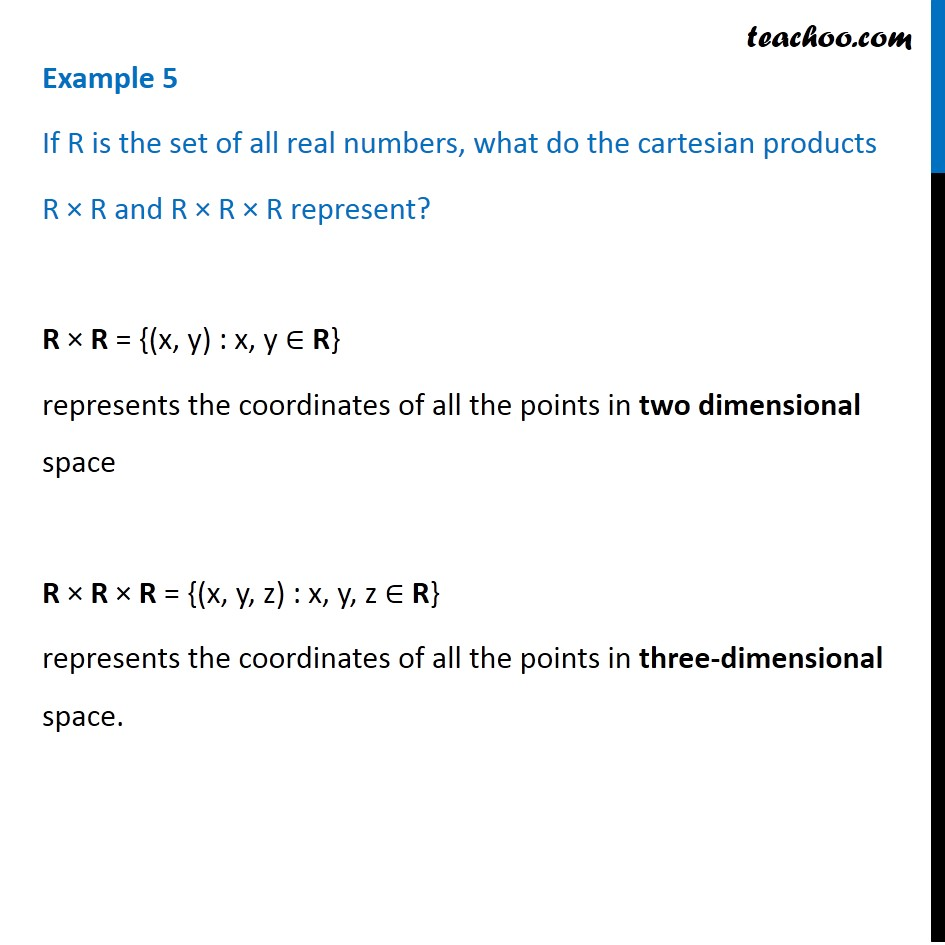Examples

Chapter 2 Class 11 Relations and Functions
Serial order wiseLearn in your speed, with individual attention - Teachoo Maths 1-on-1 Class

### Transcript

Example 5 If R is the set of all real numbers, what do the cartesian products R × R and R × R × R represent? R × R = {(x, y) : x, y ∈ R} represents the coordinates of all the points in two dimensional space R × R × R = {(x, y, z) : x, y, z ∈ R} represents the coordinates of all the points in three-dimensional space.